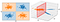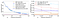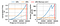# Lifting Data… and Washing Machines: Kernel Computations from Optical Random Features

The increasing size of available data and the benefit of working in high-dimensional spaces is creating an emerging need for AI-dedicated hardware. Graphics Processing Units (GPUs) have been used with great success to accelerate computations for deep learning. Yet, they have limited memory and consume large amounts of power.

At LightOn, we have developed an Optical Processing Unit (OPU) that is a scalable, memory-less, low power co-processor that computes optical random features.

Today, we introduce our work on large scale optical random features, that will be presented at the ICASSP 2020 Virtual Conference. It is the result of a collaboration between researchers at LightOn, EURECOM and Ecole Normale Supérieure. Please do not hesitate to get in touch if you are attending the virtual conference!

## A romance of many dimensionsFigure 1: The XOR problem is not linearly separable in two dimensions, but we can map it in three dimensions, the component of the additional dimension being x₃ = x₁ · x₂, and obtain perfect classification using a plane.

“Either this is madness or it is Hell.” “It is neither,” calmly replied the voice of the Sphere, “it is Knowledge; it is Three Dimensions: open your eye once again and try to look steadily.”

Flatland: A Romance of Many Dimensions, E. Abbott Abbott 1884

In Machine Learning, most of the problems are not linearly separable: we cannot discriminate classes using a simple hyperplane. For instance, the XOR problem can not be solved using a single line (Figure 1 — left). The simple yet powerful idea behind kernel methods is to map the data points into a higher dimensional space where, with high probability, they become linearly separable (Cover’s theorem). For the XOR problem we can introduce the following feature map:

mapping each point x ∈ ℝ² into the so-called feature space ℝ³, where the points become linearly separable (Figure 1 — right).
It is possible to project to feature spaces objects with more complex structures. For example text documents, where the mapping could be the counting of words into histograms: given the histograms it is possible to compare the documents and classify them.

Kernel methods  are a class of algorithms that operate in a high-dimensional, implicit feature space. An amazing property of kernels is that the classification is often performed on an infinite number of features, as long as a correlation matrix appears in the algorithm. Indeed, a kernel can be written as:

This expression is a correlation (or similarity) matrix in the projected feature space. Quite interestingly, the advantage of kernel methods is that we can choose the type of correlation without writing explicitly the function ϕ (for example radial basis function (RBF)). Indeed, in most cases, it is impossible to explicitly find a finite-dimensional feature map. Kernel methods also enjoy well established theoretical development to statistically determine the accuracy of the solution. Recent developments in kernel-based methods such as FALKON  have shown state-of-the-art accuracy on several datasets.

## Throwing the random kitchen sinks at the problem

The major drawback of kernel methods is that given n data points, there is a need to compute the correlation for all pairs of points. If we write these correlations in a matrix form, we have an n×n kernel matrix that is really expensive to compute and store when the number of data points is large. Given current computational capabilities, exact kernel methods are therefore used only for average-sized datasets — n ～10⁴ points.

Let us assume a problem with n data points of dimension D. Using traditional kernel methods we would need to compute and store a matrix of elements. If n=1.000.000, this could require . Naively inverting this matrix to perform Kernel Ridge Regression  would cost n³ operations or about an . As a result of this difficult scaling, several solutions have been proposed to overcome this limitation.

Ali Rahimi and Ben Recht developed the technique of random features [4, 5]: a distance-dependent kernel (such as the RBF) can be approximated in expectation by the dot product of randomized feature maps. The randomness is sampled from the Fourier transform of the kernel. For instance, for the RBF, we have:

where wᵢ∼N(0, 𝕀 / σ²).

Using D random features, we only need to store the data projected using random features (n×D) and the covariance matrix (D×D). Assuming for example n=1.000.000, d=1.000, D=10.000, we have reduced the memory requirement by a factor 100×. Moreover, the ridge regression solution now involves the inversion of a 10.000×10.000 matrix, instead of a matrix of size 1.000.000×1.000.000.

Thus, if the number of random features used is much lower than the number of samples, there is a substantial reduction in the complexity related to the problem. Recent work  has proven that a number of random features could be of order of

is sufficient, under certain reasonable assumptions, to obtain the same statistical accuracy of the exact Kernel Ridge Regression. In our example with n=1.000.000, we would need 14.000 random features to reach statistical optimality.

LightOn’s OPU performs the following operation at the speed of light:

where U∈ℝᴰˣᵈ in which each element is sampled from the complex Gaussian distribution, x∈ℝᵈ, and the absolute value squared is applied element-wise. The exponent can be changed numerically, leading to different kernels. In this randomized feature map, the input and output size (d and D) can scale .
We computed the kernels associated with the optical random features: they are dot product kernels, that measure the angular correlation between data points. Numerically, we can change the exponent of the feature map and, when the exponent m is even, i.e. m=2s, the formula of the kernel is:

Where C is a coefficient depending on s and i. The formula for the feature map of exponent m=2 is:

By increasing the exponent of the feature map, we measure higher-order correlations between the data points, thus obtaining a finer similarity measure, at the cost of increased risk of over-fitting.

## Sinking the error ship in a sea of dimensions

We started by using optical random features in a kernel ridge regression (KRR) setup: we projected the data using the OPU, then we performed penalized linear regression in the new space. We used the Fashion-MNIST dataset and compare optical random features of different exponents to the commonly used RBF random features. We projected the data up to dimension 10⁵ and plotted the classification error as a function of the projection dimensions (Figure 2). The exact kernel accuracies for the RBF and the Optical (m=2) kernels are reached at dimension D=100.000.Figure 2: Ridge Regression test error on Fashion MNIST for different RFs and projection dimensions D. Horizontal lines show the test error using the true kernel. Standard deviations for different seeds are negligibly small and not shown in the plot. Plot (a) compares optical RFs of degree m=2 to RBF Fourier RFs. Higher degree optical RFs are left out for better readability. The more slowly converging optical RFs for m=4 are added for larger D in plot (b).

## Bonjour Optical Transfer Learning

Convolutional neural networks (CNNs) are best suited for image classification, therefore we turned to transfer learning. We combined the power of CNNs and of the random features to increase the accuracy of a simple CNN by replacing the last dense layer of common pre-trained networks by a kernel ridge regression classifier, where the random projection is performed using the OPU.
We projected to dimension 10⁴ using the OPU. In architectures with a smaller final layer (e.g. ResNet34, d =512), we obtained an increase in accuracy of more than 2.4%. With a final layer size of the order of the projection dimension, we conserved the same accuracy (e.g. AlexNet, d = 9216). However, when the projection dimension is smaller than the size of the final layer (e.g. VGG16, d =25088), it acts as a non-linear compression, potentially decreasing the accuracy. Therefore, there is a trade-off to be found between speedup and machine learning performance.

## The light energy consumption of light computationFigure 3: Time and energy spent on computing a matrix multiplication (n,D) × (D,D). The batch size n is 3000 (solid line) or 1000 (dotted line). The OPU is compared to an NVIDIA P100 GPU.

In Figure 3, we compare the energy consumption of the OPU with respect to the GPU. The energy consumption of the optical processing is independent of the input and output dimension and is about 45 J (30W times 1.5 seconds). The GPU consumes more energy when increasing the projection dimension, up to 7× (~315 J) more than the OPU at D=56.000, where the GPU hits the memory limit.

A factor 7× in energy consumption is the difference between the energy required to lift a suitcase or a washing machine up two floors (~450J vs ~3150 J)!

“ My back still hurts.”
Laurent Daudet, CTO at LightOn, about his experience in moving a washing machine up two floors.

You can find the code used for the paper here: https://github.com/joneswack/opu-kernel-experiments

You can try to reproduce it or make your own calculations with an OPU through our LightOn Cloud. The Cloud will be available on March
31st. You can subscribe .

LightOn and LightOn Cloud support research. From March 31st you will be able to apply to the LightOn Cloud Program for Research on .

LightOnis a that develops new optical processors that considerably . open new horizons in computing and engineering fields that are facing computational limits. Interested in speeding your computations up? on ! 🌈

Follow us on Twitter at @LightOnIO , subscribe to our newsletter and register to our workshop series. We live stream, so you can join from anywhere. 🌍

# The author

Ruben Ohana is a PhD student at Ecole Normale Supérieure, INRIA & LightOn. Ruben acknowledges support from Région Ile-de-France.

# References

 Bernhard Schölkopf, Alexander J Smola, Francis Bach, et al., “Learning with kernels: support vector machines, regularization, optimization, and beyond”, MIT Press, 2002.

 Alessandro Rudi, Luigi Carratino, and Lorenzo Rosasco, “Falkon: An optimal large scale kernel method,” Advances in Neural Information Processing Systems, 2017, pp. 3888–3898.

 Murphy, K. P., “Machine Learning: A Probabilistic Perspective”, chapter 14.4.3, pp. 492-493, The MIT Press, 2012

 Ali Rahimi and Benjamin Recht, “Random features for large-scale kernel machines,” in Advances in neural information processing systems, 2008, pp. 1177–1184.

 Ali Rahimi and Benjamin Recht, “Weighted sums of random kitchen sinks: Replacing minimization with randomization in learning,” in Advances in neural information processing systems, 2009, pp. 1313–1320.

 Alessandro Rudi, and Lorenzo Rosasco, “Generalization Properties of Learning with Random Features”, Advances in Neural Information Processing Systems, 2017.

Written by

Written by

## More From Medium

Welcome to a place where words matter. On Medium, smart voices and original ideas take center stage - with no ads in sight. Watch
Follow all the topics you care about, and we’ll deliver the best stories for you to your homepage and inbox. Explore
Get unlimited access to the best stories on Medium — and support writers while you’re at it. Just \$5/month. Upgrade